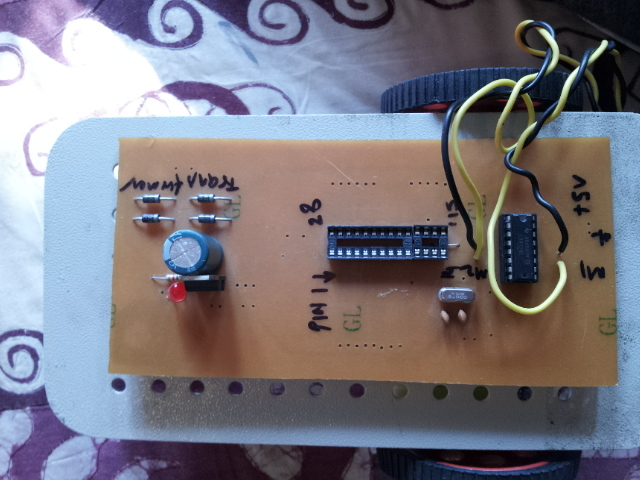# Grid solver

I want a algorithm for a grid solver bot in which i have to travel through a maze and then stop at a node and blink a led.

Hello.

One of the members of this forum, Geoff, has done a similar project with a 3pi robot and documented it on his website:

tabletoprobotics.xyz/tabletop/gridnav.html

–David

Hello sir,
I have one more question.I made a program written in c language and i need some of your help.I want to know whether this program will work on ATMEGA 8 or not.I have modified an already written program.Also i want you to help me in adding a PID program with this program and a program to blink a led at selected node.
I will also be grateful if you review my program and check it for mistakes and better corrections.
Thanks
Bot.doc (25.5 KB)

It is a very simple bot with only controller and no display and buttons.

–David

``````#include<at89x52.h>
#include<stdio.h>
/*
P0 for stepper control
P1_2 for ldr detect
*/
#define ldra P1_3
#define ldrb P1_1
#define ldrc P1_2
#define ldrd P1_4
unsigned char speed=20;
void delay()
{
unsigned char i,j;
for(i=0;i<speed;i++)
for(j=0;j<128;j++);
}
void dely(int i) //stop for 40 seconds
{
int j,k;
for(k=0;k<i;k++)
for(j=0;j<1250;j++);
}
void left(int i)
{
int k;
for(k=0;k<i;k++)
{
P0=0x11;
delay();
P0=0x33;
delay();
P0=0x22;
delay();
P0=0x66;
delay();
P0=0x44;
delay();
P0=0xCC;
delay();
P0=0x88;
delay();
P0=0x99;
delay();
}
}
void forw(int i)
{
int k;
for(k=0;k<i;k++)
{
P0=0x91;
delay();
P0=0x83;
delay();
P0=0xC2;
delay();
P0=0x46;
delay();
P0=0x64;
delay();
P0=0x2C;
delay();
P0=0x38;
delay();
P0=0x19;
delay();
}
}
void leftforwmix(int i)
{
int k;
for(k=0;k<i;k++)
{
P0=0x90;

delay();
P0=0x81;
delay();
P0=0xC0;
delay();
P0=0x43;
delay();
P0=0x60;
delay();
P0=0x22;
delay();
P0=0x30;
delay();
P0=0x16;
delay();
P0=0x90;
delay();
P0=0x84;
delay();
P0=0xC0;
delay();
P0=0x4C;
delay();
P0=0x60;
delay();
P0=0x28;
delay();
P0=0x30;
delay();
P0=0x19;
delay();

}
}
void rightforwmix(int i)
{
int k;
for(k=0;k<i;k++)
{
P0=0x09;
delay();
P0=0x91;
delay();
P0=0x03;
delay();
P0=0x82;
delay();
P0=0x06;
delay();
P0=0xC4;
delay();
P0=0x0C;
delay();
P0=0x48;
delay();
P0=0x09;
delay();
P0=0x61;
delay();
P0=0x03;
delay();
P0=0x22;
delay();
P0=0x06;
delay();
P0=0x34;
delay();
P0=0x0C;
delay();
P0=0x18;
delay();

}
}
void right(int i)
{ int k;
for(k=0;k<i;k++)
{

P0=0x88;
delay();
P0=0xCC;
delay();
P0=0x44;
delay();
P0=0x66;
delay();
P0=0x22;
delay();
P0=0x33;
delay();
P0=0x11;
delay();
P0=0x99;
delay();

}
}

void takeleft()
{
// forw(6);
right(22);
forw(20);

}
void takeroto()
{
// forw(6);
right(44);
forw(20);

}
void takeright()
{
// forw(6);
left(22);
forw(20);

}
void stop()//stop
{
while(P2_0!=0);
}
void main()
{unsigned char c,d;
int i=0,junction=0;
P0=0x00;
P1=0xFF;
P2=0xFF;
ira=1;
irb=1;
irc=1;
ird=1;
forw(13);
while(1)
{ c=P1&0x1E;
if(c==0x18)// if robot is at the center
{
c=P1&0x06;
forw(1);
}
else if(c==0x1C)// if right misalign
{
c=P1&0x06;
leftforwmix(1);
}
else if(c==0x1A)// if left misalign
{ c=P1&0x06;
rightforwmix(1);
}
else if(c==0x00)
{ junction++;
forw(10);
d=P1&0x18;
switch(junction)
{
case 2:speed=15;takeright();break;
case 4:speed=15;dely(5000);takeleft();break;
case 7:speed=15;takeright();break;
case 8:speed=15;dely(5000);break;
case 9:speed=15;takeright();break;
case 13:speed=15;takeright();break;
case 17:speed=8;P0=0x00;stop();break;
}
}
else
{
forw(1);
}
//P0=0x00;
}
}``````The LED blink program is not working.I have deleted the program here in the code.can anyone give me a working led blinking code?